### 第一: 美图虽好，不是首选。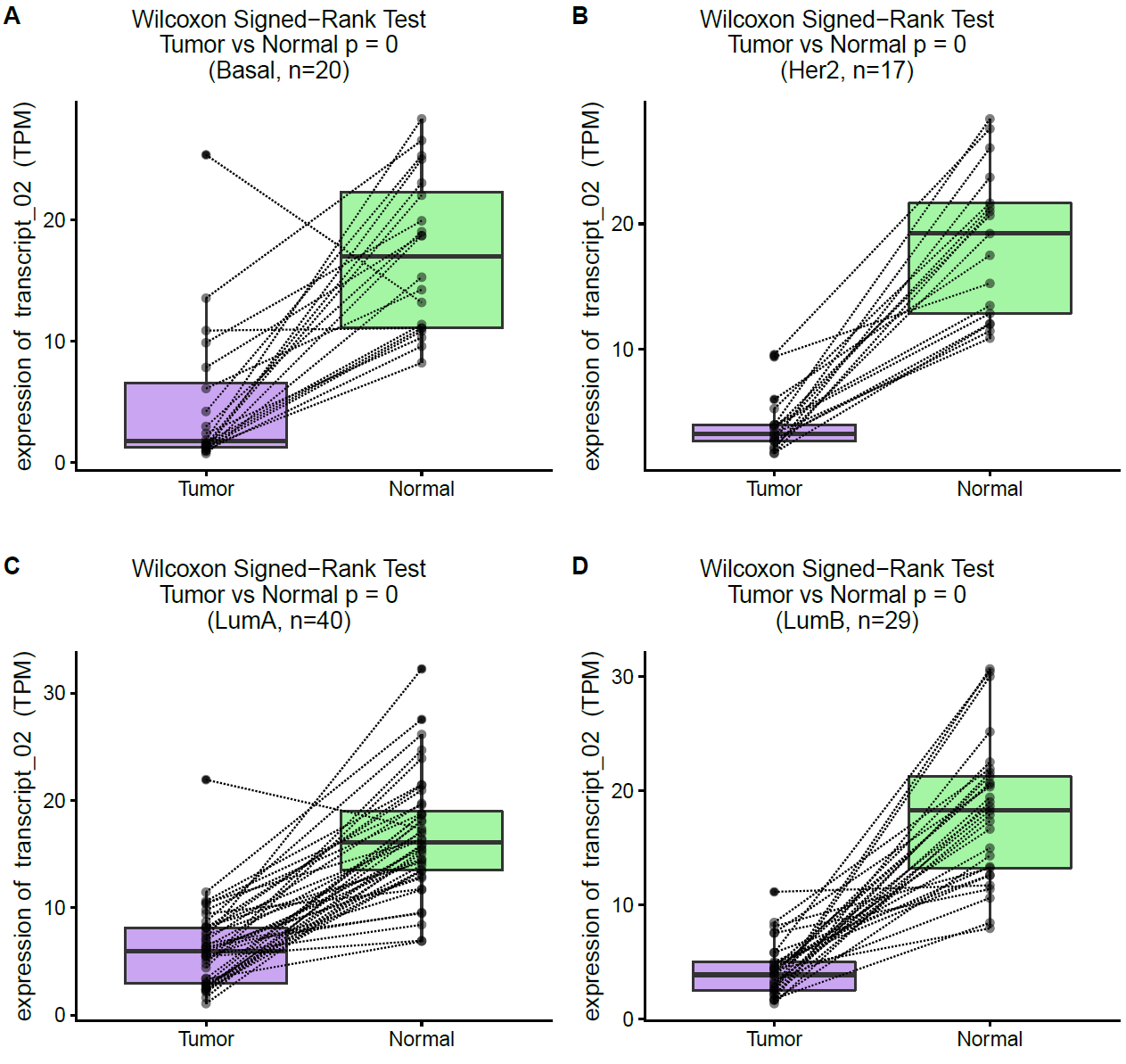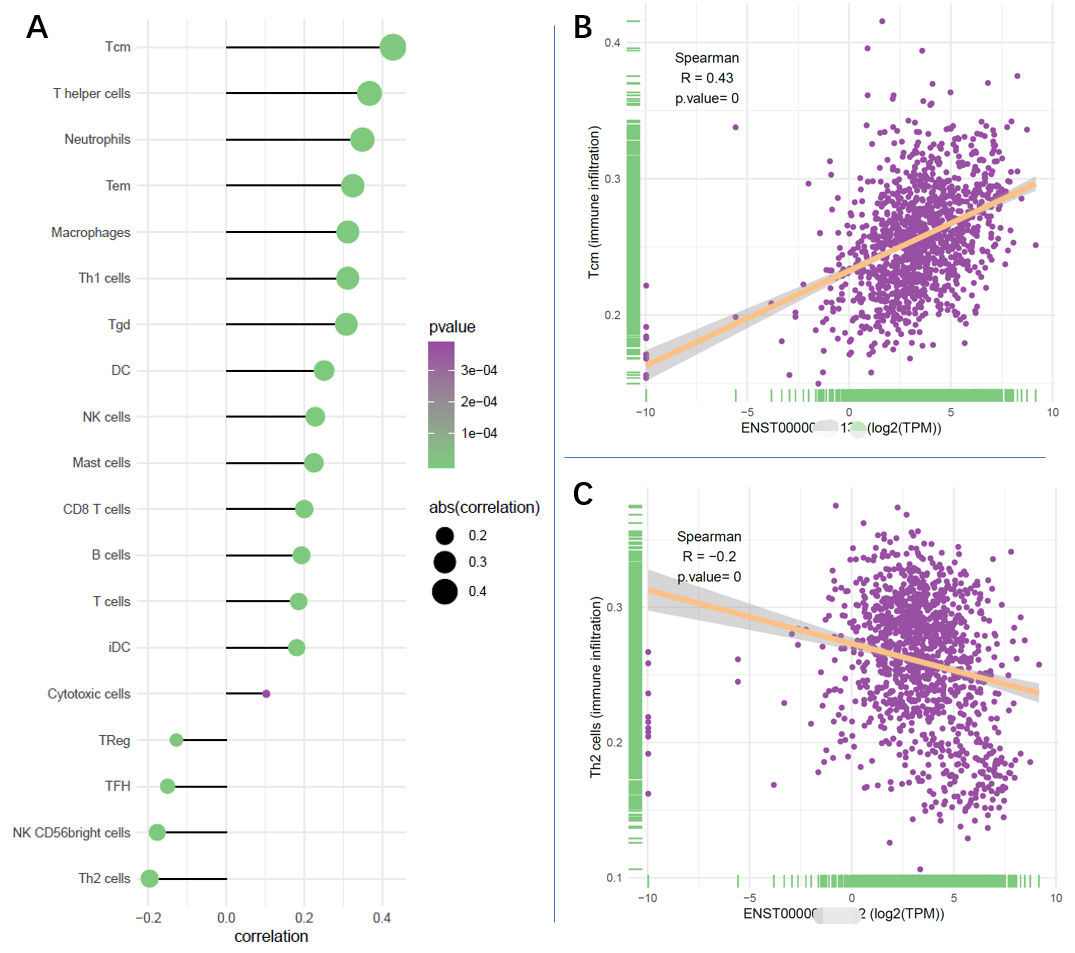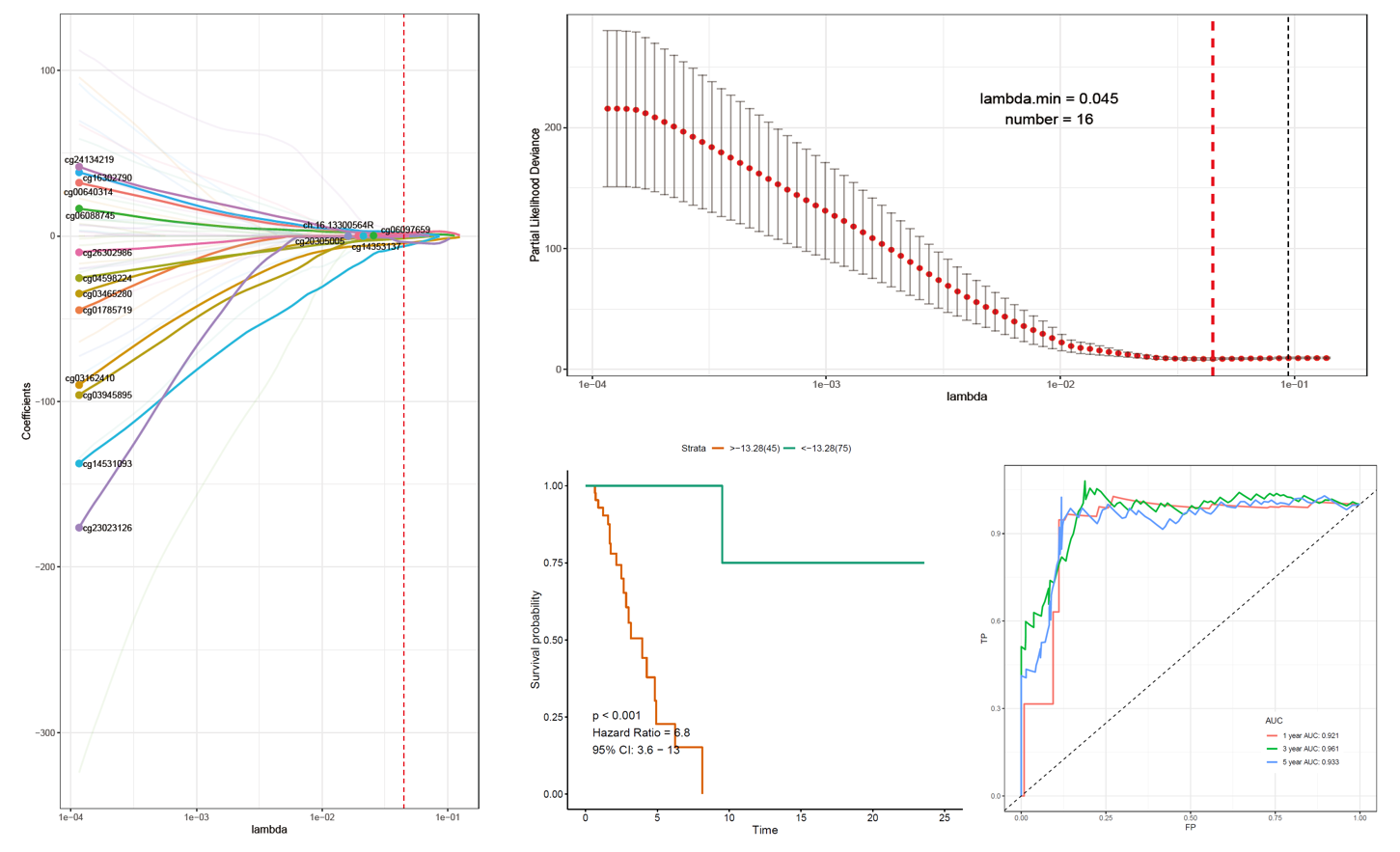### 第二：好的课程，要让学员觉得自己牛逼，而不是让学员觉得讲师牛逼。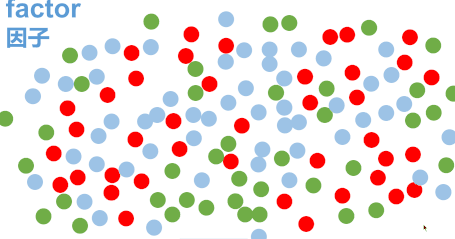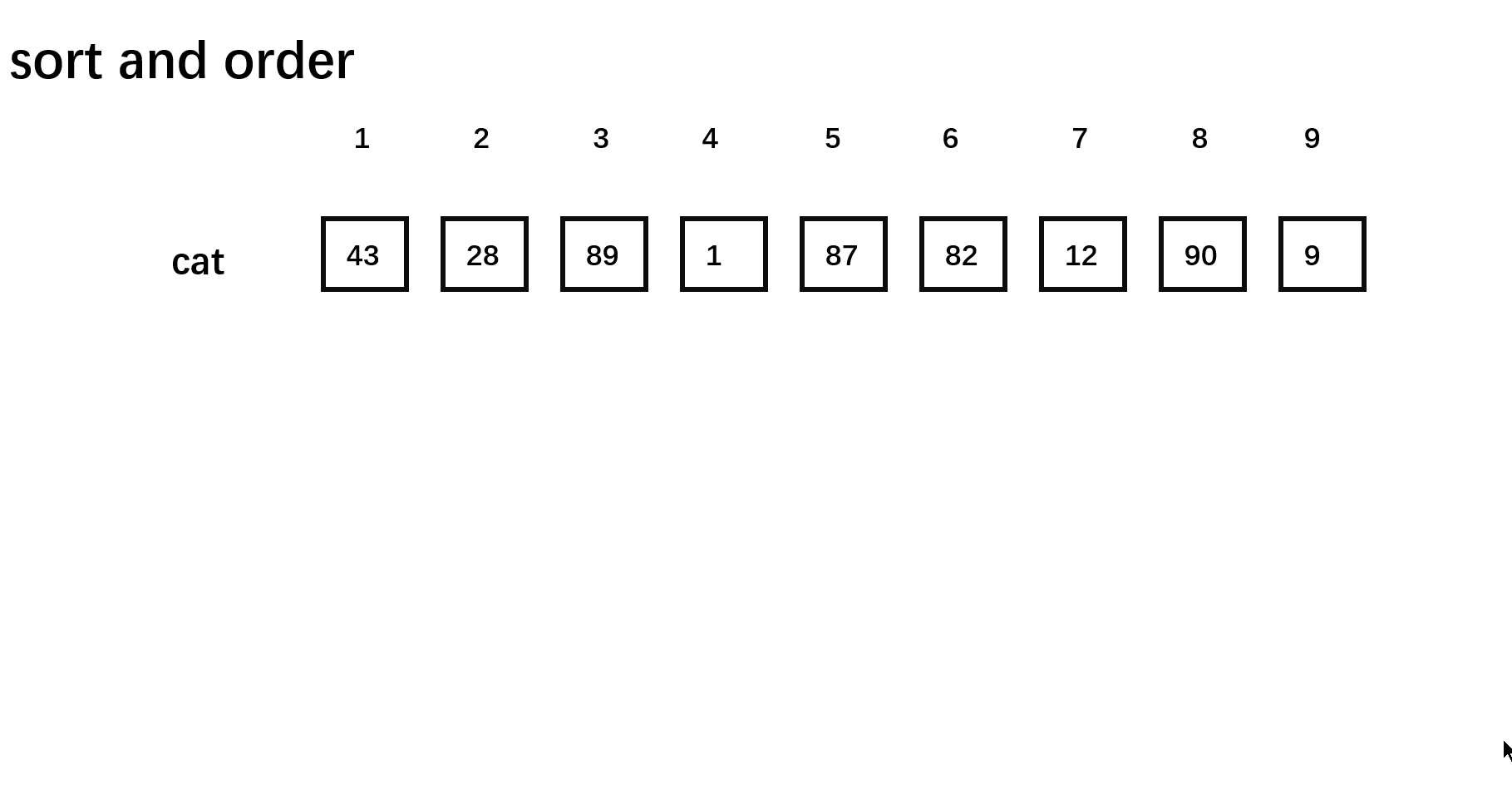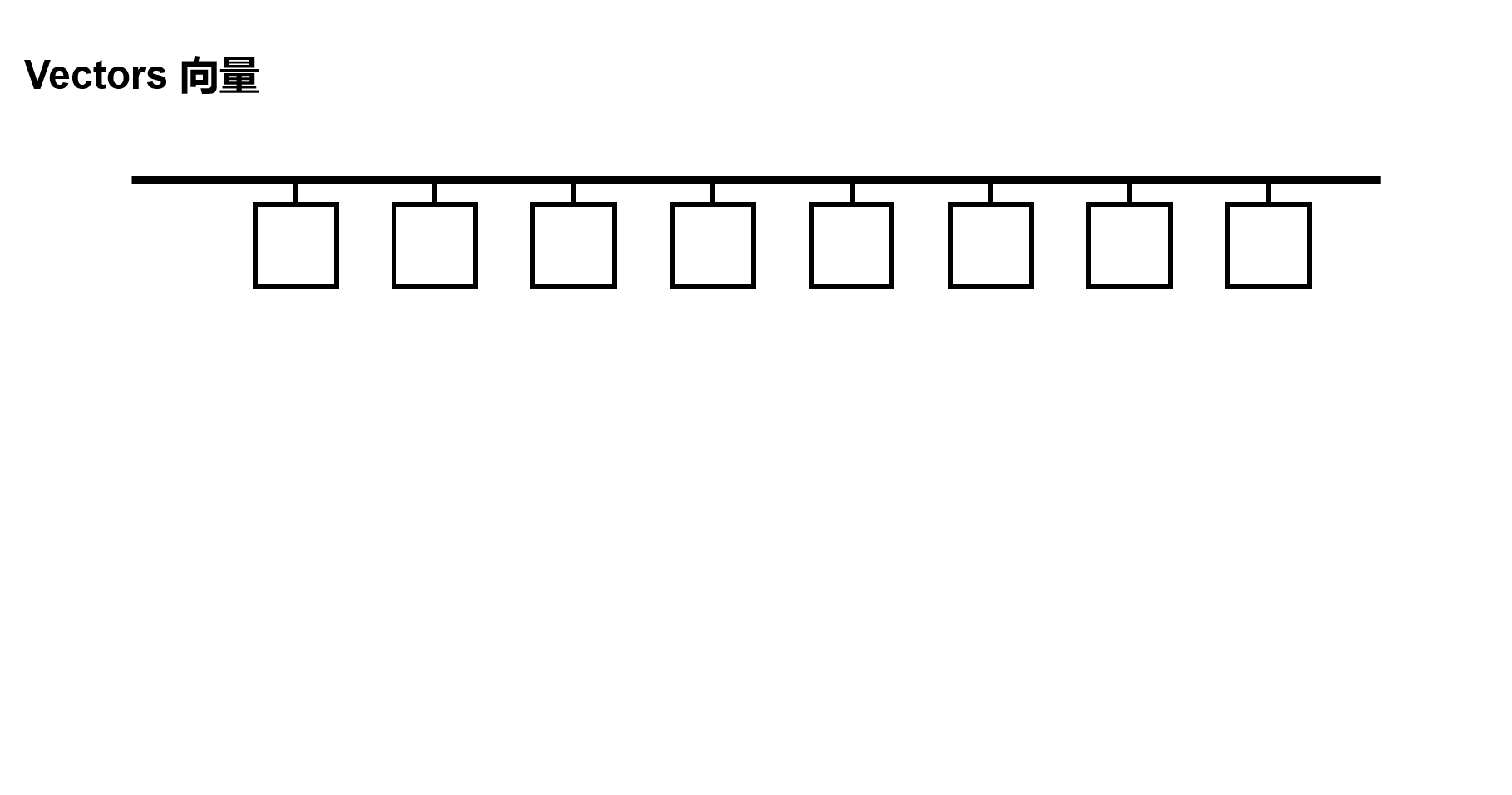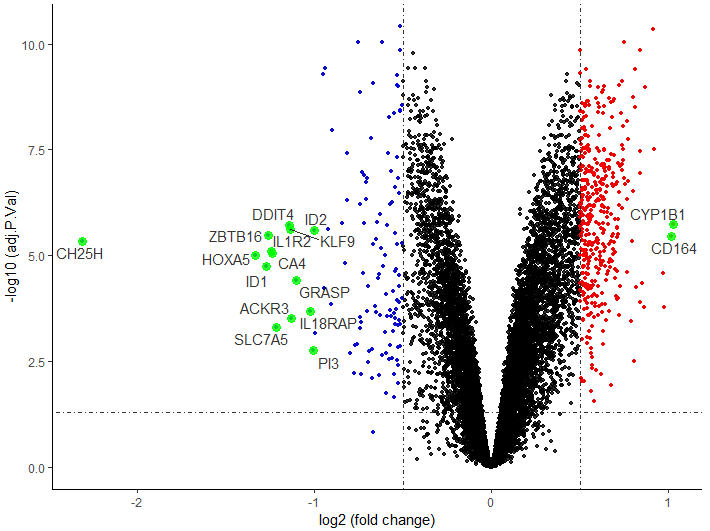• 我们教过大家如何用检索解决R语言报错
• 如果通过检索获取想要的资源和教程
• 如何用检索查询本领域的数据，无论你是心内科还是神内科，都有数据可用
• 我们还展示过一次无敌人肉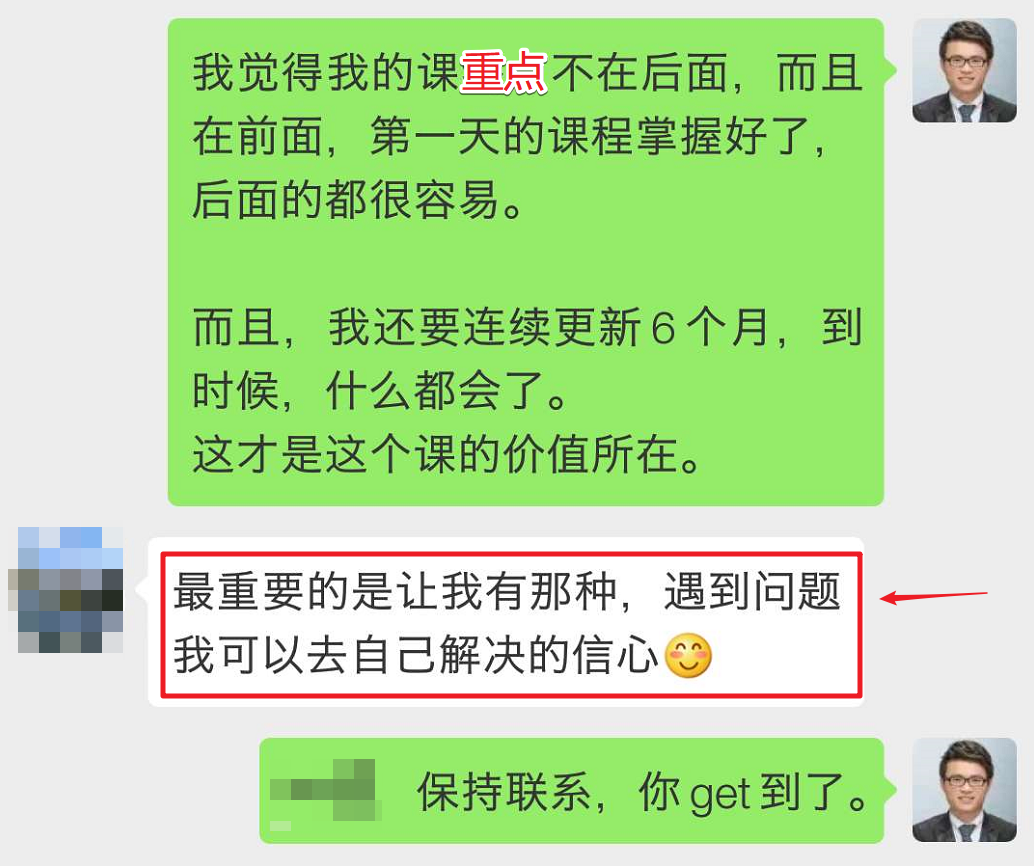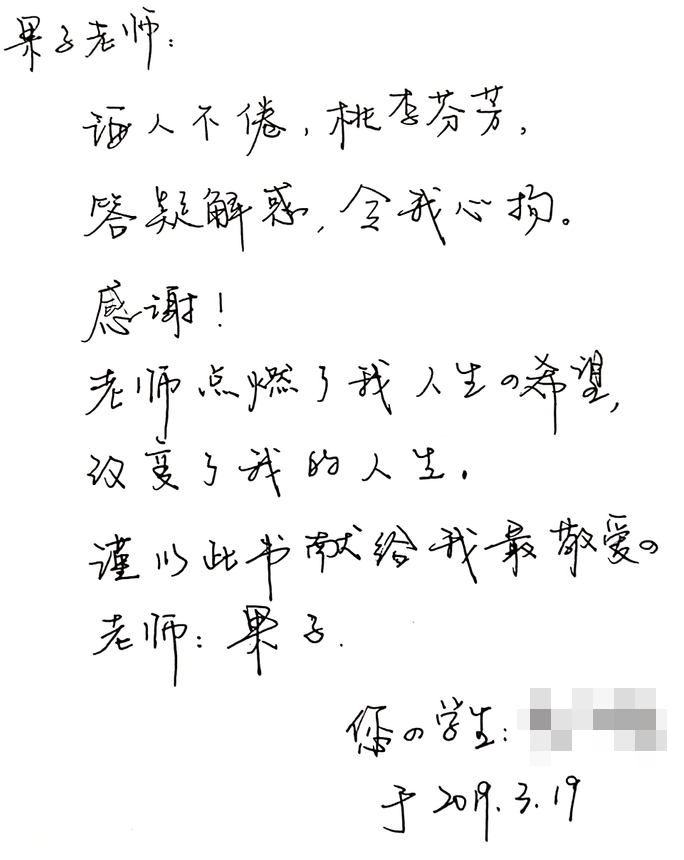### 本次会议的通用课表(每次会作改动)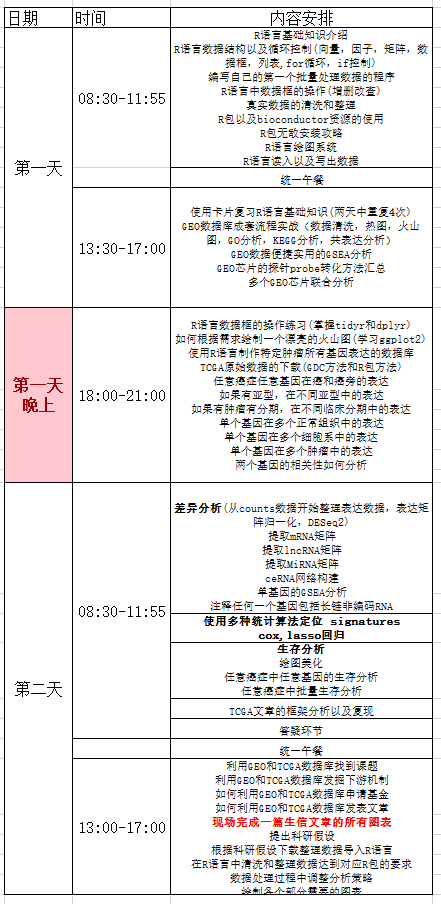### 会议费用：

3400元/人
(按交费先后顺序安排座次，注册费包含会场、教材、午餐、视频课程等费用，不含住宿费）

### 如果有意愿，给我发邮件报名：

guoshipeng2008@126.com

1、姓名：
2、性别：
3、邮箱：
4、手机：
5、发票抬头：如不确定，先留空。
6、纳税人识别号或统一社会信用代码：如不确定，先留空。
7、是否预订住宿：如需预订住宿请注明，一般默认住宿时间是从会议前一晚到会议最后一天中午退房，如果有特殊需要请注明。
8、备注：备注说明写在此，如有多人参加，请将姓名、性别、邮箱、手机在上面分别单独列出，备注中写明一位主要联系人。

### 额外说明

]]>
<![CDATA[视频小教程_如何画出没有教程的图？]]> https://codingsoeasy.com/archives/digheatmap https://codingsoeasy.com/archives/digheatmap#comments Jan 17, 2020, 3:38:48 AM （本次操作有配套的视频教程，在果子学生信公众号回复“果子对角线”自行获取，和代码一起以project的形式分享）

### 果子唠嗑

ggplot2版本，excel版本，complexheatmap版本，base plot版本。

• 如何创建数据框？
• 如何融合多个数据框？
• 如何实现数据的宽长转换？
• 如何调整x轴label的顺序？
• 多张图如何拼接？
• ggplot2怎么用？
• 多边形怎么画？
• 如何把大需求拆解成可完成的小步骤？
• R语言中批量操作神器lapply怎么用？

### 以下是正文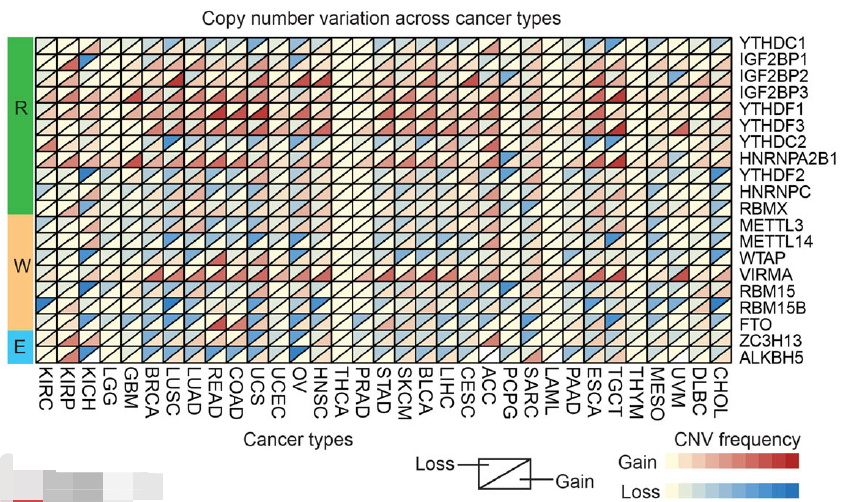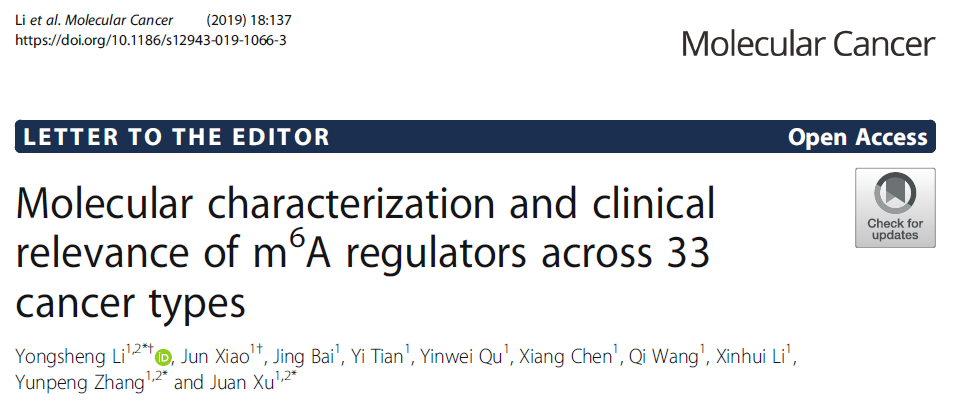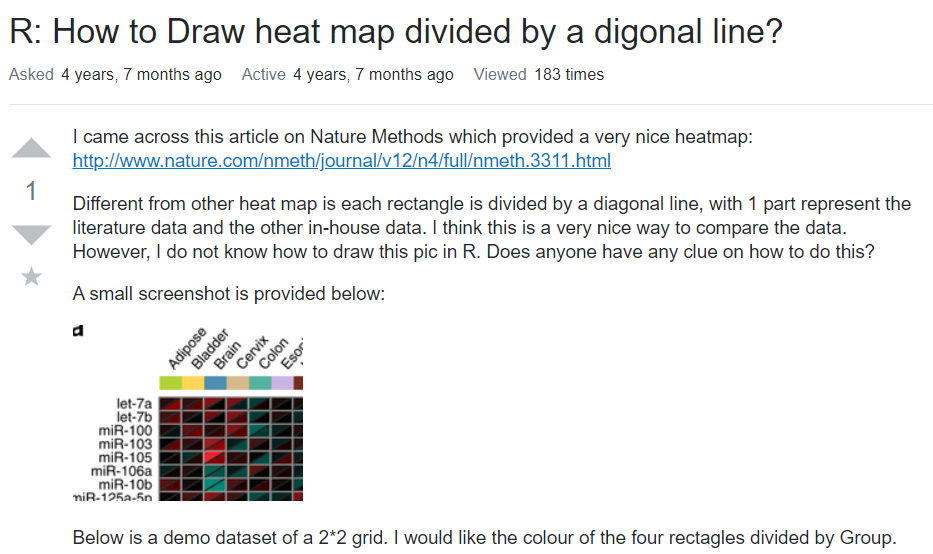``````d=data.frame(p1=rep(LETTERS[1:2],times=2,each=2),
p2=rep(LETTERS[4:5],times=4),
value=c(.2,.4,.56,.32,.7,.16,.12,.71),
group=rep(c("A1","B1"),each=4))

x=as.numeric(d\$p1)
y=as.numeric(d\$p2)

plot(1,xlim=c(1,length(unique(x))+1),ylim=c(1,length(unique(y))+1),
type="n",bty="n",xaxt="n",yaxt="n",xlab="",ylab="")

for(i in 1:nrow(d)) {
if(d\$group[i]=="A1") polygon(x[i]+c(0,1,1),y[i]+c(0,0,1),col=gray(d\$value[i]))
if(d\$group[i]=="B1") polygon(x[i]+c(0,1,0),y[i]+c(0,1,1),col=gray(d\$value[i]))
}

axis(1,at=sort(unique(x))+.5,labels=levels(d\$p1),lty=0)
axis(2,at=sort(unique(y))+.5,labels=levels(d\$p2),lty=0)
``````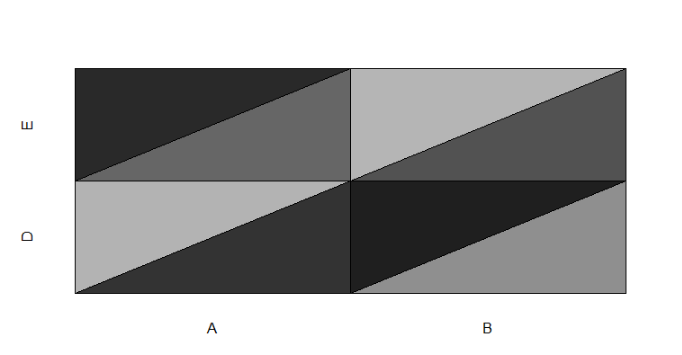base plot版本的对角线热图

(果子:此时，我还不会写图层，尽管有这样的思想，但是没有趁手的工具)

``````## 下三角数据
cone1 = data.frame(x = c(1,2,2),
y = c(1,1,2))
library(dplyr)
library(ggplot2)
## 下三角画图
cone1 %>%
ggplot(aes(x=x, y=y)) +
geom_polygon(fill="#213c18")
``````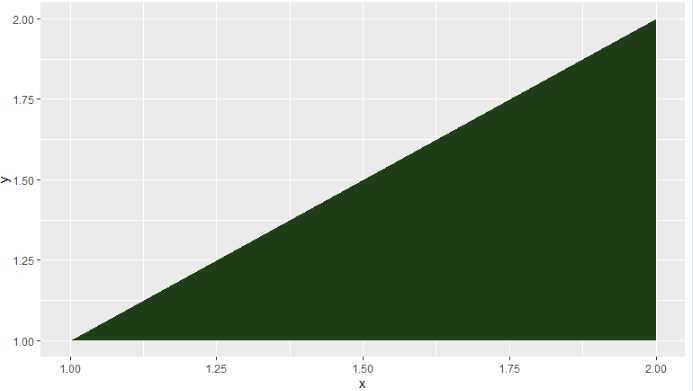``````## 上三角数据
cone2 = data.frame(x = c(1,1,2),
y = c(1,2,2))
## 上三角画图
cone2 %>%
ggplot(aes(x=x, y=y)) +
geom_polygon(fill = "#668c6f")
``````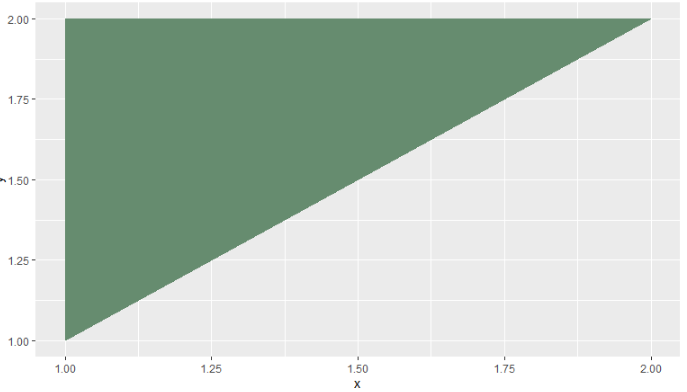``````cone1 %>%
ggplot(aes(x=x, y=y)) +
geom_polygon(fill="#213c18")+
geom_polygon(data=cone2, fill = "#668c6f")
``````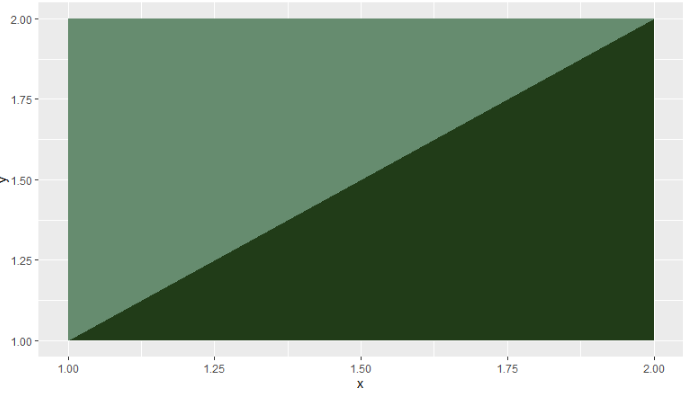``````trianle <- function(a,b,type="up"){
## 单个下三角数据的函数
trianle_down <- function(a,b){
data.frame(x=c(0,1,1)+a,
y= c(0,0,1)+b,
group=paste0(a,"_",b),
stringsAsFactors = F)
}
## 单个上三角数据的函数
trianle_up <- function(a,b){
data.frame(x=c(0,0,1)+a,
y= c(0,1,1)+b,
group=paste0(a,"_",b),
stringsAsFactors = F)
}
### 批量产生上三角的数据
if(type=="up"){
data <- do.call(rbind,lapply(1:b, function(i){
do.call(rbind,lapply(1:a,trianle_up,i))
}))
}
### 批量产生下三角的数据
if(type=="down"){
data <- do.call(rbind,lapply(1:b, function(i){
do.call(rbind,lapply(1:a,trianle_down,i))
}))
}
return(data)
}
``````

``````updata <- trianle(33,20,"up")
downdata <- trianle(33,20,"down")
``````

updata的数据是这样的，每一个框线里面都是一个三角形的数据，group那一列是为了`geom_polygon`画图需要。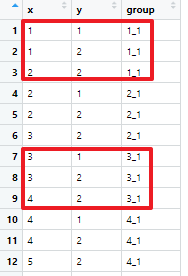``````library(ggplot2)
updata %>%
ggplot(aes(x=x, y=y)) +
geom_polygon(aes(group=group,fill=group))+
theme(legend.position = "none")
``````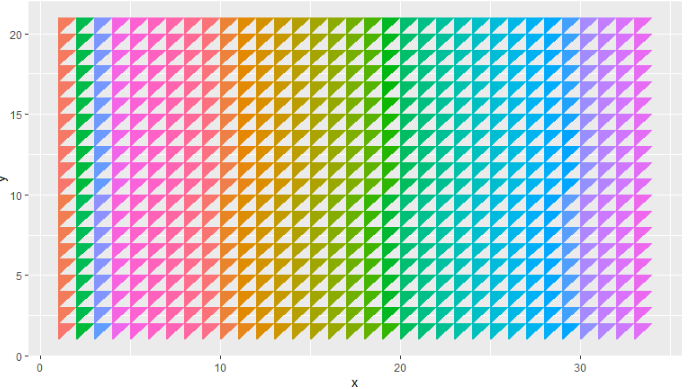``````downdata %>%
ggplot(aes(x=x, y=y)) +
geom_polygon(aes(group=group,fill=group))+
theme(legend.position = "none")
``````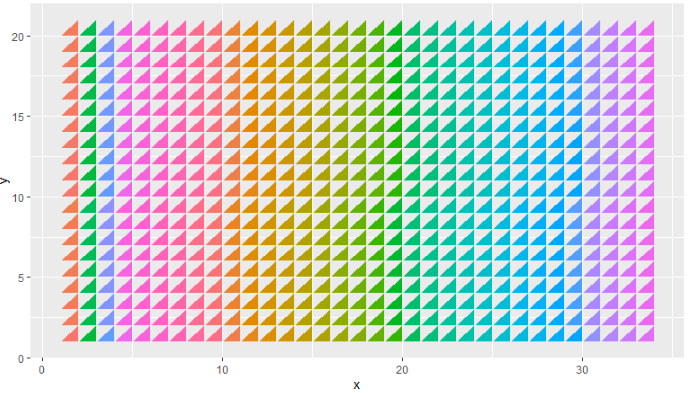``````dd <- data.table::fread("easy_input_amp.txt",data.table = F)
``````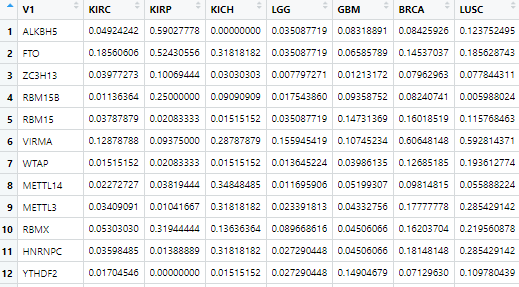``````library(dplyr)
library(tidyr)
data1 <- dd %>%
## 数据变长
pivot_longer(-1,
names_to = "type",
values_to = "exp") %>%
rename(gene=V1)
``````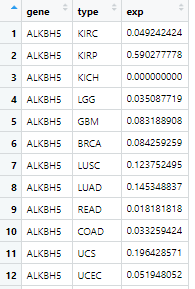``````data1\$gene <- factor(data1\$gene,
levels = c("ALKBH5","FTO",
"HNRNPA2B1","HNRNPC", "IGF2BP1", "IGF2BP2","IGF2BP3","RBMX","YTHDC1","YTHDC2","YTHDF1","YTHDF2","YTHDF3",
"METTL14","METTL3","RBM15","RBM15B","VIRMA","WTAP","ZC3H13"),
ordered = F)
``````

``````data1 <- data1 %>%
mutate(a=as.numeric(as.factor(type)),
b=as.numeric(gene)) %>%
mutate(group = paste0(a,"_",b)) %>%
as.data.frame() %>%
inner_join(updata,by="group")
``````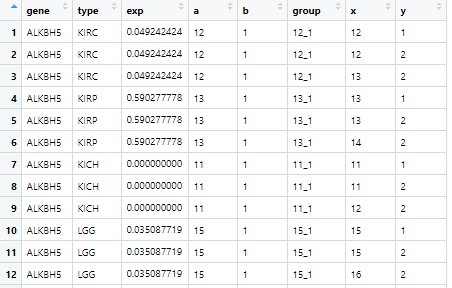``````library(ggplot2)
data1 %>%
ggplot(aes(x=x, y=y)) +
geom_polygon(aes(group=group,fill=exp))+
theme(legend.position = "none")
``````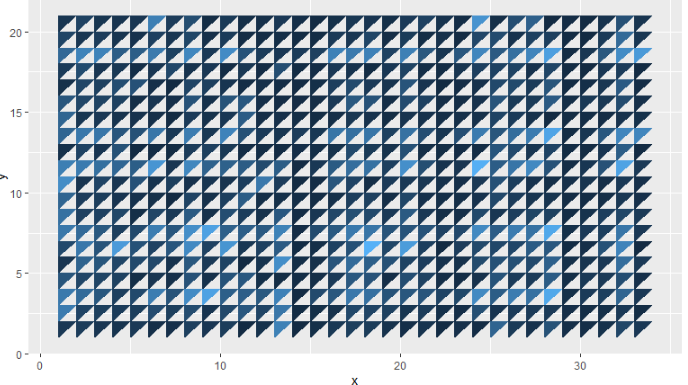``````dd <- data.table::fread("easy_input_del.txt",data.table = F)
data2 <- dd %>%
pivot_longer(-1,
names_to = "type",
values_to = "del") %>%
rename(gene=V1)

data2\$gene <- factor(data2\$gene,
levels = c("ALKBH5","FTO",
"HNRNPA2B1","HNRNPC", "IGF2BP1", "IGF2BP2","IGF2BP3","RBMX","YTHDC1","YTHDC2","YTHDF1","YTHDF2","YTHDF3",
"METTL14","METTL3","RBM15","RBM15B","VIRMA","WTAP","ZC3H13"),
ordered = F)
data2 <- data2 %>%
mutate(a=as.numeric(as.factor(type)),
b=as.numeric(as.factor(gene))) %>%
mutate(group = paste0(a,"_",b)) %>%
as.data.frame() %>%
inner_join(downdata,by="group")

data2 %>%
ggplot(aes(x=x, y=y)) +
geom_polygon(aes(group=group,fill=del))+
theme(legend.position = "none")
``````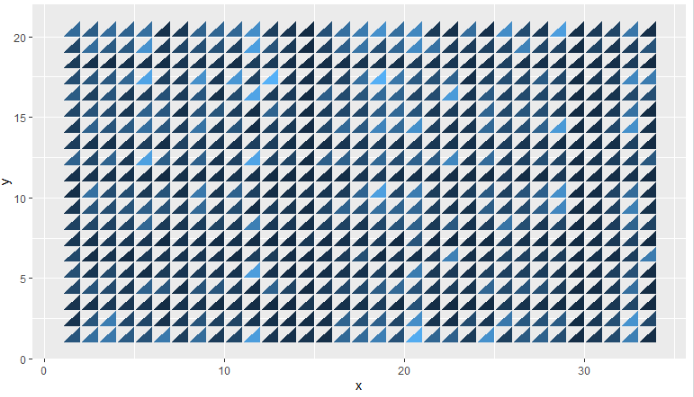``````options("repos"=c(CRAN="https://mirrors.tuna.tsinghua.edu.cn/CRAN/"))
if(!require("ggnewscale")) install.packages("ggnewscale",update = F,ask = F)
library(ggnewscale)
``````

``````## x轴文字
xlabels <- data1 %>%
filter(y==1) %>%
distinct(type,.keep_all = T) %>%
arrange(x) %>%
pull(type)
## y轴文字
ylabels <- data1 %>%
filter(x==1) %>%
distinct(gene,.keep_all = T) %>%
arrange(y) %>%
pull(gene) %>%
as.character()
``````

``````red  <- "#AB221F"
blue <- "#3878C1"
``````

``````p1 <- ggplot(data1,aes(x=x, y=y)) +
## 画出上三角
geom_polygon(data = data1,aes(x=x, y=y, group=group,fill=exp),color="black") +
## 上三角用表达值来配色
## 神技能，清空aes
new_scale_fill() +
## 画出下三角
geom_polygon(data = data2,aes(x=x, y=y, group=group,fill=del),color="black") +
## 下三角用表达值来配色
## 调整x轴
scale_x_continuous(limits = c(1, NA), expand = c(0,0),breaks = c(1:33)+0.5,labels=xlabels) +
## 调整y轴
scale_y_continuous(limits = c(1, NA), expand = c(0,0),breaks = c(1:20)+0.5,labels=ylabels) +
## 定主题
theme(axis.title.x=element_blank(),
axis.title.y=element_blank(),
axis.text.x = element_text(angle = 90, hjust = 1))+
## legend置于底部
theme(legend.position="bottom")+
theme(plot.margin = margin(0.5,0.01,0.5,0.01, "cm"))
p1
``````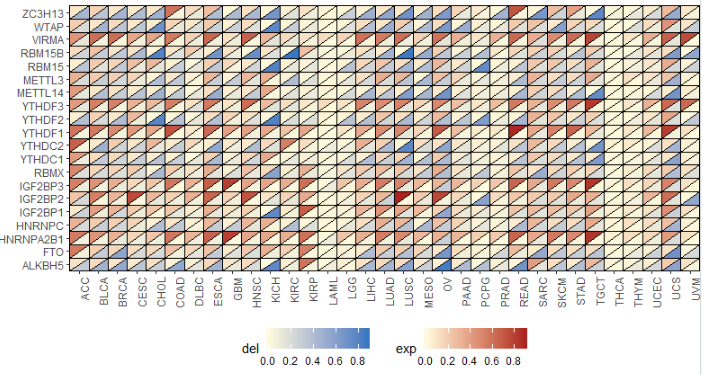``````data <- data.frame(
x = rep(c(1,1,2,2),3),
y = c(1,3,3,1,3,14,14,3,14,21,21,14),
)

p2 <- ggplot(data,aes(x=x, y=y)) +
geom_polygon(aes(x=x, y=y, group=type,fill=type),color="black",alpha = 0.5)+
scale_fill_manual(values = c(red,nake,blue))+
## 打标签
geom_text(data=data.frame(x=1.5,y=c(2,8.5,17.5)),
angle = 90,
size=4)+
scale_x_continuous(limits = c(1, NA), expand = c(0,0))+
scale_y_continuous(limits = c(1, NA), expand = c(0,0)) +
theme(axis.title=element_blank(),
axis.text = element_blank(),
axis.ticks = element_blank())+
theme(legend.position="none")+
theme(plot.margin = margin(0.5,0.1,0.5,0.01, "cm"))
p2
``````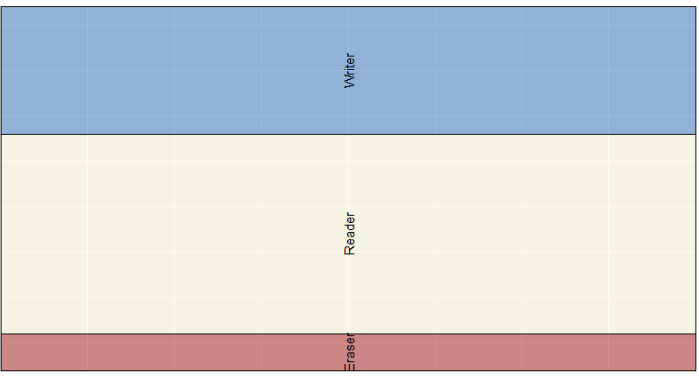``````library(cowplot)
plot_grid(p1,p2,align = "h", axis = "tb",nrow = 1, rel_widths = c(33, 1))
``````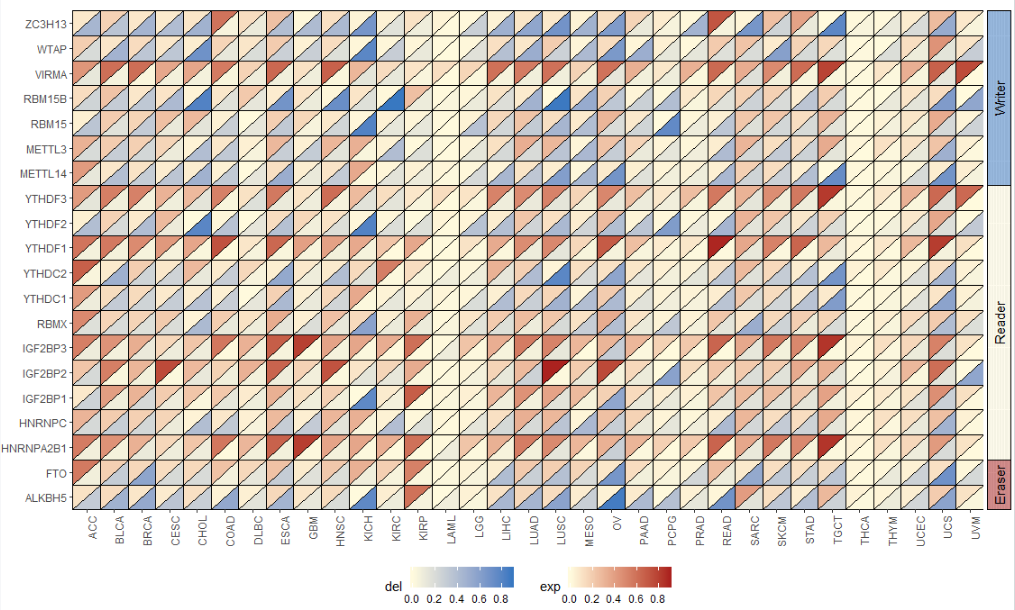``````options("repos"=c(CRAN="https://mirrors.tuna.tsinghua.edu.cn/CRAN/"))
if(!require("patchwork")) install.packages("patchwork",update = F,ask = F)
library(patchwork)
p1 + p2 +
plot_layout(widths = c(33, 1))
``````

### 果子备注

]]>
<![CDATA[视频小教程_R语言中的批量操作]]> https://codingsoeasy.com/archives/apply https://codingsoeasy.com/archives/apply#comments Jan 17, 2020, 3:34:09 AM （本次操作有配套的视频教程，在果子学生信公众号回复“果子爱批量”自行获取，和代码一起以project的形式分享）

8秒完成2万个基因的生存分析，人人都可以！

### 1.lapply

``````lapply(as.list(1:4), log2)
``````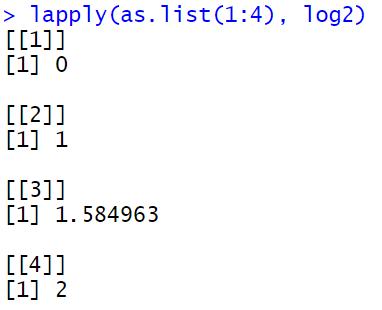``````lapply(1:4, log2)
``````

lapply的厉害之处在于，他作用的function可以自己写，那么这样一来就千变万化了。

``````lapply(1:4, function(x){
x^2 +2
})
``````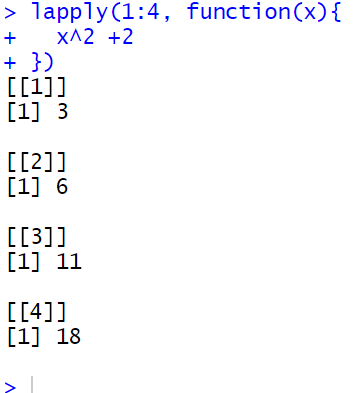### 2.do.call

do.call中的函数，需要能够接受多个参数。

cbind(), rbind(),c()都有这个特点。

``````sum(..., na.rm = FALSE)
c(...)
cbind(..., deparse.level = 1)
rbind(..., deparse.level = 1)
``````

lapply是list在前，函数在后

``````lapply(1:4, log2)
``````

do.call是函数在前，列表在后

``````dd <- lapply(1:4, log2)
``````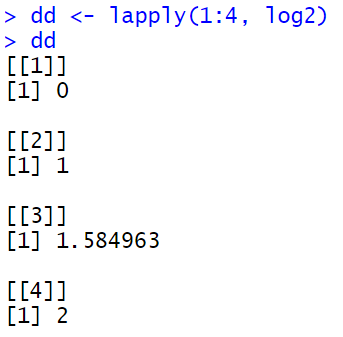``````do.call(c,dd)
``````

`````` 0.000000 1.000000 1.584963 2.000000
``````

### 3.lapply 和do.call 连用

``````dd <- lapply(LETTERS[1:6], function(i){
paste0(i,1:10)
})
``````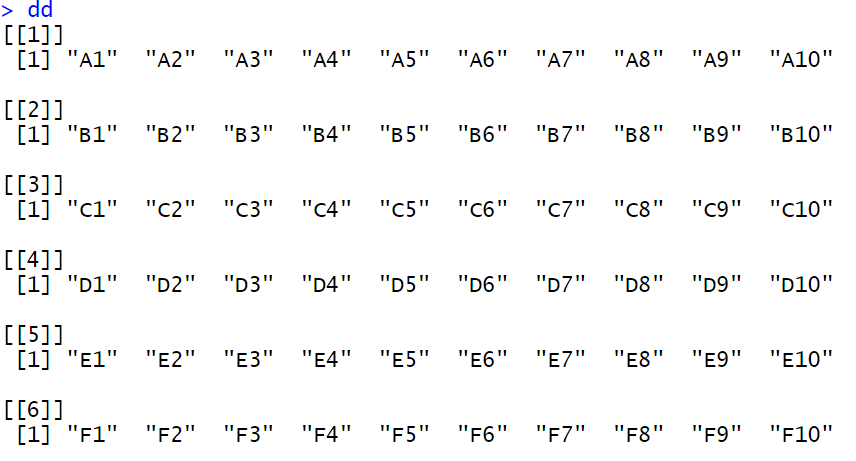``````do.call(rbind,dd)
``````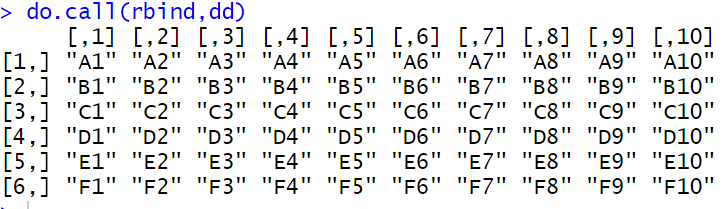``````do.call(cbind,dd)
``````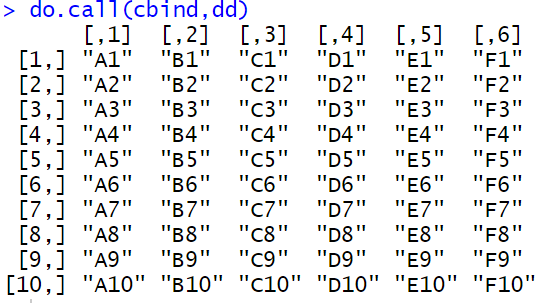R语言中性价比最高的函数以及最贵的函数

``````union(x, y)
intersect(x, y)
setdiff(x, y)
setequal(x, y)
``````

### 4.Reduce

`Reduce`函数就是一个解决方案。

Reduce函数实现多个数据框批量合并

### 5.推荐阅读

lapply并行化处理

R语言中的批量分组操作

]]>
<![CDATA[果子的TCGA教程汇总，持续更新。]]> https://codingsoeasy.com/archives/tcga https://codingsoeasy.com/archives/tcga#comments Jan 4, 2020, 7:04:25 PM ​首先看这几个帖子：

TGCA数据的标准化以及差异分析

TCGA真实数据下的批量生存分析

TCGA提取非编码RNA并完成下游分析

GTF文件有什么用啊？别的不谈，最起码能提lncRNA

Y叔推荐的这个图有毒！

TCGA的模型预测模型构建：

TCGA构建临床预测模型的大坑

Reduce函数实现多个数据框批量合并

]]>
<![CDATA[果子的GEO芯片分析教程汇总, 持续更新。]]> https://codingsoeasy.com/archives/geo https://codingsoeasy.com/archives/geo#comments Jan 4, 2020, 6:51:36 PM 这是个导航帖，可以有效解决芯片分析的大部分问题。内容抵得上一个GEO的培训班。

GEO分析有三个限速环节

#### 第二，平台获取

skr！GEO芯片数据的探针ID转换

GEO芯片中的NM_，NR_开头的识别号如何转换成基因名称？

#### 第三，序列比对

GEO芯片分析的倒数第2个关卡被没有了

Limma求差异基因构建矩阵的两种方式

GEO的样本名称太多而且排序不规则，你们都是手动分组的么？

GEO芯片分析中的大坑，差异基因完全相反！

GEO芯片如果超过了两组，也可以一次搞定差异分析

GEO芯片中配对样本如何做差异分析

GEO芯片中多个探针对应一个基因，是求平均值还是保留最大值？

QQ-plot

K均值聚类预测未知分类信息

K邻近算法来分析芯片数据

]]>
<![CDATA[dplyr需要掌握的6个函数]]> https://codingsoeasy.com/archives/dplyr https://codingsoeasy.com/archives/dplyr#comments Jan 4, 2020, 2:21:45 AM dplyr是我十分喜欢的包，这里面需要掌握的函数并不多，但是很有用。

### 数据集介绍

• 萼片长度(Sepal.Length)
• 萼片宽度(Sepal.Width)
• 花瓣长度(Petal.Length)
• 花瓣宽度(Petal.Width)

• Iris Setosa（山鸢尾）
• Iris Versicolour（杂色鸢尾）
• Iris Virginica（维吉尼亚鸢尾

``````library(dplyr)
``````

### 1.select 选择列

select()函数的使用： 筛选的是列 最普通最常用的的方法， 按照列名筛选列， 比如只筛选Petal.Length,Petal.Width两个变量

``````df_select <- select(iris, Petal.Length, Petal.Width)
``````

• starts_with，
• ends_with，
• contains，
• matches，
• one_of，
• everything

``````df_select_1 <- select(iris, starts_with("Petal"))
``````

``````df_select_2 <- select(iris, ends_with("Width"))
``````

``````df_select_3 <- select(iris, contains("etal"))
``````

``````df_select_4 <- select(iris, matches(".t."))
``````

``````df_select_5 <- select(iris, everything())
``````

``````vars <- c("Petal.Length", "Petal.Width")
df_select_6 <- select(iris, one_of(vars))
``````

### 2.mutate 增加列

mutate()函数的使用，新增列 注意他不仅仅可以随意增加列，还可以对已有列数据变换并添加为新的列， 举例子，添加一个新的列new，并且new是Sepal.Length和Sepal.Width的乘积

``````df_mutate <- mutate(iris, new = Sepal.Length * Sepal.Width)
``````

### 3.filter 选择行

filter()函数的使用 筛选的是行 按照已定条件对行做过滤，类似标准函数subset, 需要注意的是，可以添加多个标准，多个标准之间以逗号隔开， 举例子，筛选Species是setosa的数据

``````df_filter_1 <- filter(iris, Species == "setosa")
``````

``````df_filter_2 <- filter(iris, Sepal.Length > 5,Species == "setosa")
``````

``````df_filter_3 <- filter(iris, Sepal.Length > 5, Species %in% c("setosa","versicolor"))
``````

### 4.arrange 行排序

arrange()函数的使用 #对数据进行排序，类似标准函数order： 按照Sepal.Length从小到大排序

``````df_arrange_1 <- arrange(iris, Sepal.Length)
``````

``````df_arrange_2 <- arrange(iris, Sepal.Length, desc(Sepal.Width))
``````

### 5.distinct 行去重

distinct()函数的使用, 他的作用是以某个列为指标来把去重行， 用法如下

``````distinct(.data, ..., .keep_all = FALSE)
``````

data就是我们的数据框，三个点的地方填入的是要去重的指标，一般是列名，.keep_all 这个参数指定的是，其余列是否需要保留 我们举个例子来说明一下 我们以Species为指标来去重，因为他只包含三个分类，所以最终只有三个元素留下，此时把.keep_all = F，那么其他列就会被去掉， 只剩下Species这一列

``````test <- distinct(iris,Species,.keep_all = F)
``````
``````> test
Species
1     setosa
2 versicolor
3  virginica
``````

``````test <- distinct(iris,Species,.keep_all = T)
``````

``````> test
Sepal.Length Sepal.Width Petal.Length Petal.Width    Species
1          5.1         3.5          1.4         0.2     setosa
2          7.0         3.2          4.7         1.4 versicolor
3          6.3         3.3          6.0         2.5  virginica
``````

### 7.管道符号

]]>
<![CDATA[group_by和summrise连用后，分组计算就很方便！]]> https://codingsoeasy.com/archives/groupby https://codingsoeasy.com/archives/groupby#comments Jan 4, 2020, 12:15:48 AM 关于分组计算这个话题，我在写过的那些帖子里面，经常用到的就是`group_by`联合`summarise` 比如多探针求最大值，多甲基化位点求平均值，TCGA中miRNA求最大值 GEO芯片中多个探针对应一个基因，是求平均值还是保留最大值？ 批量读入TCGA的miRNA数据(注意细节) R语言学习路上的忆苦思甜 凡是重复的，全部删掉，一个都不留！ 从零开始学技能，以数据集合upset图为例。

`group_by`按照某一列把数据框分成多个组我是知道的，但是`summarise`我就比较疑惑了，因为他们家还有`sammarise_all`, 而且哪些函数能用在 `summarise`中，我也没有总结过，这次就准备来解决这个事情。

``````set.seed(1119)
data <- data.frame(ID=rep(LETTERS,each=3),
sample1=sample(seq(10,1000),78),
sample2=sample(seq(10,100),78),
sample3=sample(seq(20,500),78))
``````

`group_by``summarise`都存在于`dplyr`这个包中，所以需要先加载R包。

``````library(dplyr)
``````

``````data %>%
group_by(ID) %>%
summarise(n())
``````
``````# A tibble: 26 x 2
ID    `n()`
<fct> <int>
1 A         3
2 B         3
3 C         3
4 D         3
5 E         3
6 F         3
7 G         3
8 H         3
9 I         3
10 J         3
``````

``````table(data\$ID)
``````
``````A B C D E F G H I J K L M N O P Q R S T U V W X Y Z
3 3 3 3 3 3 3 3 3 3 3 3 3 3 3 3 3 3 3 3 3 3 3 3 3 3
``````

``````data %>%
group_by(ID) %>%
summarise(n=n())
``````
``````# A tibble: 26 x 2
ID        n
<fct> <int>
1 A         3
2 B         3
3 C         3
4 D         3
5 E         3
6 F         3
7 G         3
8 H         3
9 I         3
10 J         3
``````

``````data %>%
group_by(ID) %>%
summarise(sample1=max(sample1))
``````
``````# A tibble: 26 x 2
ID    sample1
<fct>   <int>
1 A         990
2 B         759
3 C         752
4 D         872
5 E         672
6 F         905
7 G         649
8 H         918
9 I         579
10 J         611
``````

``````data %>%
group_by(ID) %>%
summarise_at("sample1",max)
``````

``````mean(): the mean AKA the average
sd(): the standard deviation, which is a measure of spread
min() and max(): the minimum and maximum values respectively
IQR(): Interquartile range
sum(): the sum
n(): a count of the number of rows/observations in each group
``````

``````data %>%
group_by(ID) %>%
summarise(sample1=max(sample1),
sample2=max(sample2))
``````
``````# A tibble: 26 x 3
ID    sample1 sample2
<fct>   <int>   <int>
1 A         990      84
2 B         759      89
3 C         752      95
4 D         872      93
5 E         672      79
6 F         905      91
7 G         649      78
8 H         918      48
9 I         579      80
10 J         611      94
``````

``````data %>%
group_by(ID) %>%
summarise_at(c("sample1","sample2"),max)
``````

``````data %>%
group_by(ID) %>%
summarise(sample1=max(sample1),
sample2=min(sample2))
``````
``````# A tibble: 26 x 3
ID    sample1 sample2
<fct>   <int>   <int>
1 A         990      33
2 B         759      26
3 C         752      19
4 D         872      54
5 E         672      30
6 F         905      37
7 G         649      10
8 H         918      12
9 I         579      66
10 J         611      17
``````

``````data %>%
group_by(ID) %>%
summarise(sample1=max(sample1),
sample2=max(sample2),
sample3=max(sample3))
``````

`summarise_at`函数也是可以的。

``````data %>%
group_by(ID) %>%
summarise_at(c("sample1","sample2","sample3"),max)
``````

``````data %>%
group_by(ID) %>%
summarise_all(max)
``````
``````# A tibble: 26 x 4
ID    sample1 sample2 sample3
<fct>   <int>   <int>   <int>
1 A         990      84     443
2 B         759      89     181
3 C         752      95     413
4 D         872      93     287
5 E         672      79     471
6 F         905      91     371
7 G         649      78     491
8 H         918      48     476
9 I         579      80     484
10 J         611      94     392
``````

``````data %>%
group_by(ID) %>%
summarise_all(mean)
``````
``````# A tibble: 26 x 4
ID    sample1 sample2 sample3
<fct>   <dbl>   <dbl>   <dbl>
1 A        663.    53.7    261.
2 B        521     54.7    124.
3 C        476.    47.3    334
4 D        442.    74.3    257.
5 E        554     49.7    411
6 F        671.    56.3    222.
7 G        345.    50.3    397.
8 H        638.    35.3    259.
9 I        474.    72.3    316
10 J        323.    61.3    298
``````

``````data %>%
group_by(ID) %>%
summarise_all(list(max,min,mean))
``````
``````# A tibble: 26 x 10
ID    sample1_fn1 sample2_fn1 sample3_fn1 sample1_fn2 sample2_fn2 sample3_fn2 sample1_fn3 sample2_fn3 sample3_fn3
<fct>       <int>       <int>       <int>       <int>       <int>       <int>       <dbl>       <dbl>       <dbl>
1 A             990          84         443         405          33          53        663.        53.7        261.
2 B             759          89         181         130          26          94        521         54.7        124.
3 C             752          95         413          47          19         233        476.        47.3        334
4 D             872          93         287          57          54         232        442.        74.3        257.
5 E             672          79         471         346          30         326        554         49.7        411
6 F             905          91         371         268          37          69        671.        56.3        222.
7 G             649          78         491          89          10         278        345.        50.3        397.
8 H             918          48         476         206          12          25        638.        35.3        259.
9 I             579          80         484         349          66          71        474.        72.3        316
10 J             611          94         392          49          17         237        323.        61.3        298
``````

``````data %>%
group_by(ID) %>%
summarise_all(list(max = max,min = min,mean = mean))
``````

``````# A tibble: 26 x 10
ID    sample1_max sample2_max sample3_max sample1_min sample2_min sample3_min sample1_mean sample2_mean sample3_mean
<fct>       <int>       <int>       <int>       <int>       <int>       <int>        <dbl>        <dbl>        <dbl>
1 A             990          84         443         405          33          53         663.         53.7         261.
2 B             759          89         181         130          26          94         521          54.7         124.
3 C             752          95         413          47          19         233         476.         47.3         334
4 D             872          93         287          57          54         232         442.         74.3         257.
5 E             672          79         471         346          30         326         554          49.7         411
6 F             905          91         371         268          37          69         671.         56.3         222.
7 G             649          78         491          89          10         278         345.         50.3         397.
8 H             918          48         476         206          12          25         638.         35.3         259.
9 I             579          80         484         349          66          71         474.         72.3         316
10 J             611          94         392          49          17         237         323.         61.3         298
``````

``````data <- data.frame(ID=rep(LETTERS,each=3),
sample1=sample(seq(10,1000),78),
sample2=sample(seq(10,100),78),
sample3=sample(letters,78,replace = T))
``````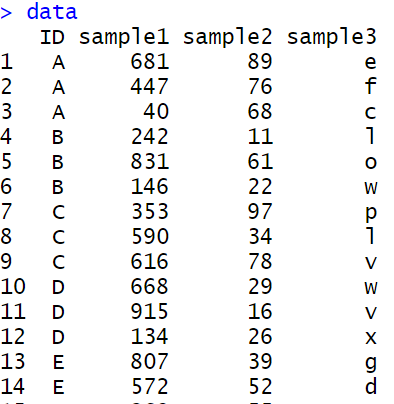这个数据的有一列不是数字，是字母，如果我想分组对所有列求和怎么办？通常的写法是这样的

``````data %>%
group_by(ID) %>%
summarise_all(max)
``````

``````Error in Summary.factor(c(4L, 5L, 2L), na.rm = FALSE) :
‘max’ not meaningful for factors
``````

``````data %>%
group_by(ID) %>%
summarise_if(is.numeric,max)
``````
``````# A tibble: 26 x 3
ID    sample1 sample2
<fct>   <int>   <int>
1 A         681      89
2 B         831      61
3 C         616      97
4 D         915      29
5 E         807      55
6 F         500      98
7 G         822      67
8 H         804      94
9 I         871     100
10 J         876      83
``````

]]>
<![CDATA[30分钟的教程写了13年，这回正则表达式有救了!]]> https://codingsoeasy.com/archives/regex https://codingsoeasy.com/archives/regex#comments Jan 4, 2020, 12:10:18 AM 关于正则表达式

``````ATG([ATCG]{3})+(TGA|TAG|TAA)
``````

R语言的正则表达式是让我头疼的，所以我干脆就没有去学。一件事情，只要不是刚需，就有拖延的可能性。后来男神Hadley Wickham 写了一个R包`stringr`使得流程简化，里面规范了正则表达式相关函数的名称。1. 查找：Detect pattern，确定这个模式有没有
2. 定位：Locate pattern， 返回模式起止位置
3. 取回：Extract pattern, 返回模式匹配到的条目
4. 替换：Replace pattern，替换匹配的模式，返回替换后的结果

#### 学习字符串处理的R包stringr

``````strings <- c(
"apple",
"219 733 8965",
"329-293-8753",
"Work: 579-499-7527; Home: 543.355.3679"
)
``````

#### 1.正则表达式中的三个括号

``````[]
{}
()
``````

#### 2.编写第一个正则表达式

``````pattern <- "([1-9][0-9]{2})[- .]([0-9]{3})[- .]([0-9]{4})"
``````

``````[1-9][0-9]{2}
[0-9]{3}
[0-9]{4}
``````

``````pattern <- "([1-9][0-9]{2})[- .]([0-9]{3})[- .]([0-9]{4})"
``````

#### 3.用str_view函数学习正则表达式

``````str_view(strings, pattern)
``````

``````str_detect(strings, pattern)
``````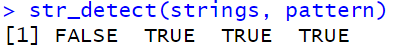结合str_view的结果，表示，2，3，4确实匹配到了结果。 2.定位 `str_locate`，从strings中检测能否和pattern匹配，返回匹配的起止位置

``````str_locate(strings, pattern)
``````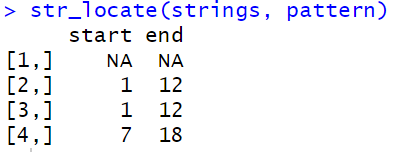3.取回 这个又分为三个层面,层层递进 第一，`str_subset`返回的是匹配到的原始条目

``````str_subset(strings, pattern)
``````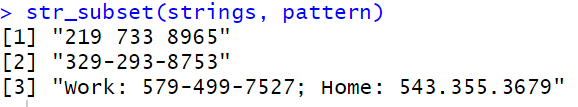第二，`str_extract`返回的是匹配到的模式

``````str_extract(strings, pattern)
``````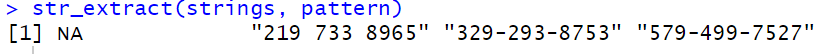第三，`str_match`返回的是数据框 第一列是str_extract的数据，后面依次是括号中的内容，模式中有多少个`()`,就返回多少列。本例中有三对小括号。

``````str_match(strings, pattern)
``````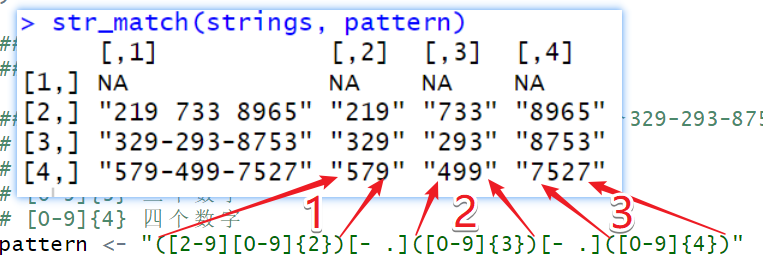4.替换 `str_replace`从strings中能够精确匹配pattern的内容，并替换为''XXX-XXX-XXXX''(此处自定义)

``````str_replace(strings, pattern, "XXX-XXX-XXXX")
``````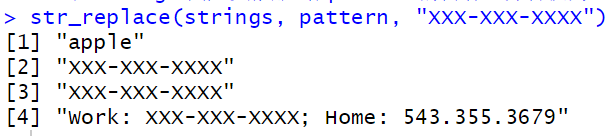#### 4.使用正则表达式来提取GEO基因symbol

(这部分内容以前写过，我复制了过来，复制自己已发布的内容，也算是抄袭) 以下这个GEO的样本名称，处理和对照夹杂，如何获取样本名称呢？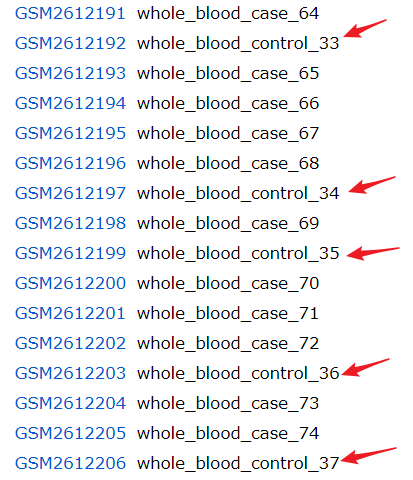``````data <- data.table::fread("gsm_group.txt",data.table = F,header = F)
names(data) <- c("GSM","group")
``````

``````library(dplyr)
library(tidyr)
separate(group,into = c("drop","group"),sep = "_blood_") %>%
select(-drop) %>%
separate(group,into = c("group","drop"),sep = "_") %>%
select(-drop)
``````

``````？
+
*
``````

``````library(stringr)
group <- str_match(data\$group,"whole_blood_(.*)_.*")
``````

``````whole_blood_(.*)_.*
``````

• 先出现`whole_blood_`
• 再出现一串字符`control`
• 后面跟着下划线`_`
• 最后跟着一串字符结束`33`

• 第一部分是，`whole_blood_`
• 第二部分是任意个数的字符`.*`
• 第三部分是下划线`_`,
• 第四部分是任意个数的字符`.*`

#### 5.匹配编码区域CDS

``````ATG([ATCG]{3})+(TGA|TAG|TAA)
``````

``````ATG([ATCG]{3})+(TGA|TAG|TAA)
``````

#### 6.转义

``````'
*
?
+
|
``````

``````str_split("a.b.c",pattern = ".")
``````

``````[]
 "" "" "" "" "" ""
``````

``````str_split("a.b.c",pattern = "\\.")
``````

``````[]
 "a" "b" "c"
``````

]]>
<![CDATA[我喜欢的gather快要被淘汰了，好在来了更好的宽长转换工具！]]> https://codingsoeasy.com/archives/pivot https://codingsoeasy.com/archives/pivot#comments Dec 24, 2019, 12:56:49 AM 果子唠嗑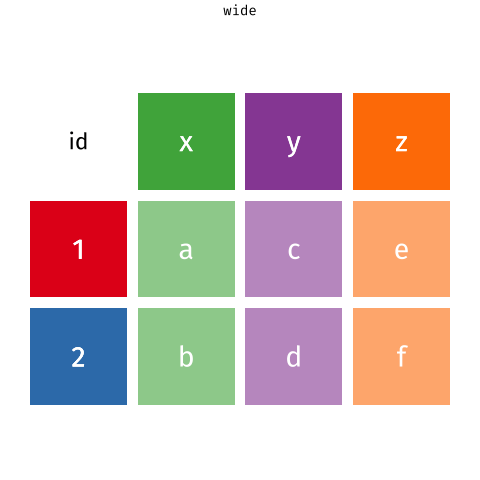#### 什么是长数据，什么是宽数据

``````load("gathe_data.Rdata")
``````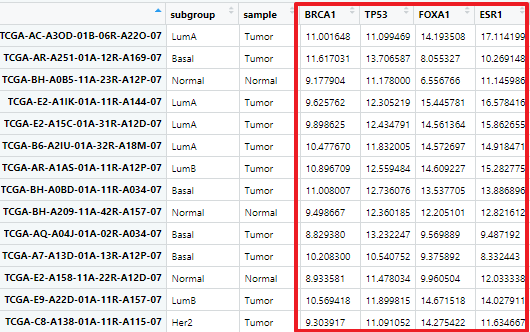宽长是相对的，取决于你要把他变成什么数据。如果我们要把他变成长数据(行数更多)，那么此刻这个数据就是宽数据。

``````library(tidyr)
data <- exprSet %>%
gather(gene,expression,3:6)
``````

exprSet就是刚才的数据，我们现在要把3:6列变成两列，数值那一列的列名是expression，3:6 列的名称所形成的列名是gene。我们看看现在的数据，他变成了4832行，4列的数据框。行数变多，就是长数据。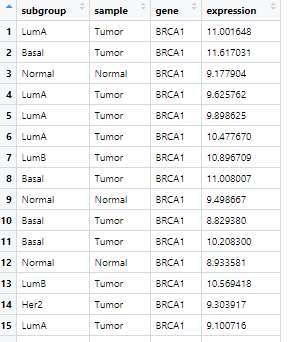#### pivot_longer和pivot_wider

``````library(tidyr)
data <- exprSet %>%
pivot_longer(cols=3:6,
names_to= "gene",
values_to = "expression")
``````

• cols 指定哪几列是要合并在一起的，数值，字符串都可以。这里用的是3:6列
• names_to 指定这几列的列名变成一列后新的名字，我没用gene来表示
• values_to 指定3:6列合并后其内容形成新的一列，他的列名是什么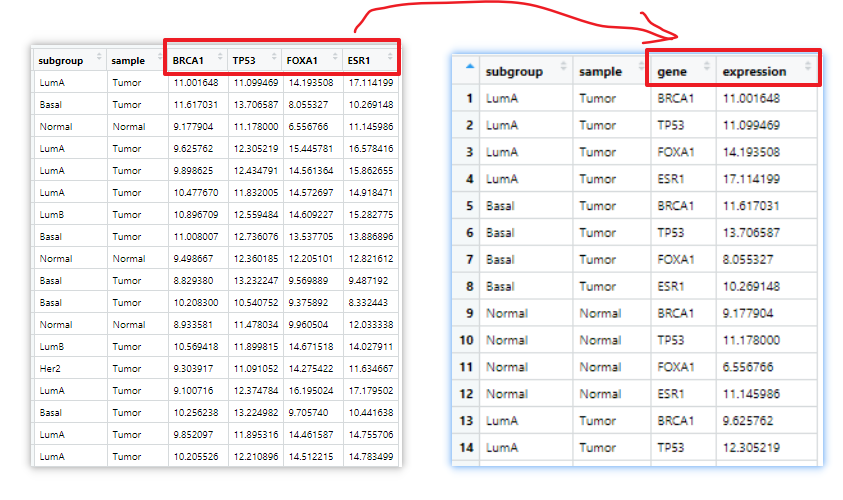#### 数据变长究竟有什么用？

``````library(ggplot2)
ggplot(exprSet,aes(x=sample,y=ESR1,fill=sample))+
geom_boxplot()
``````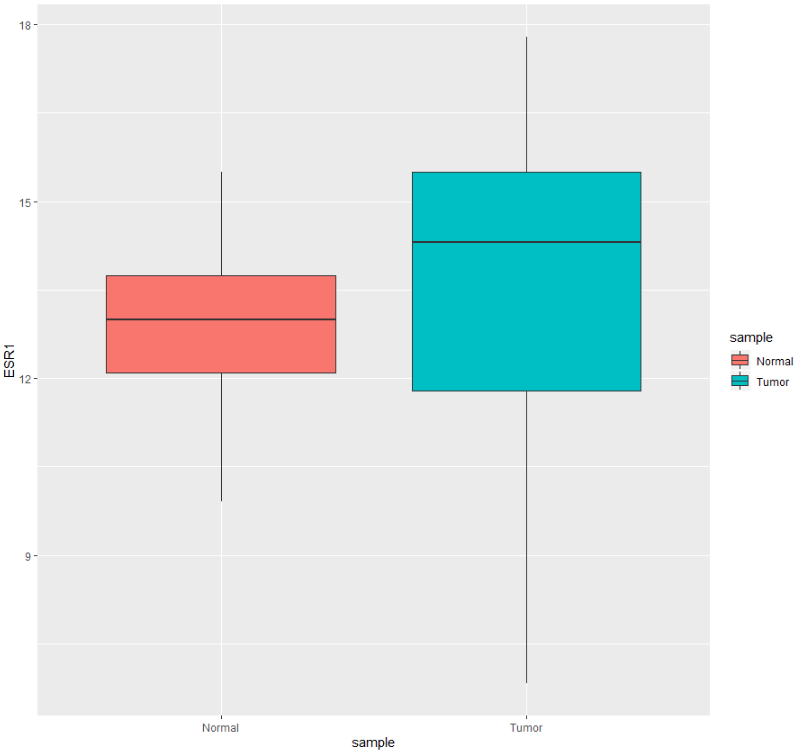``````library(ggplot2)
ggplot(data,aes(x=gene,y=expression,fill=sample))+
geom_boxplot()
``````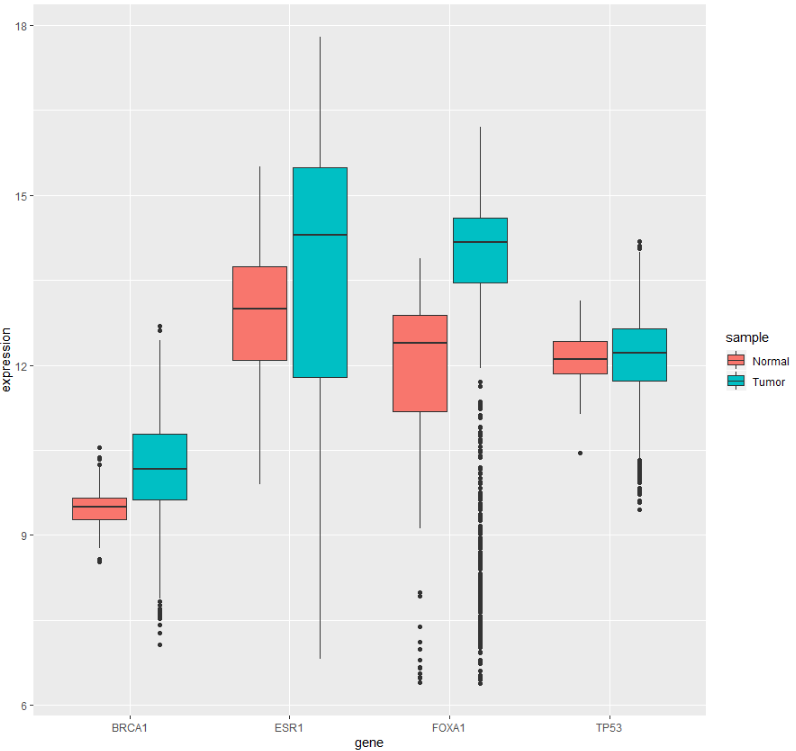如果用分面还可以实现另外的效果

``````library(ggplot2)
ggplot(data,aes(x=sample,y=expression,fill=sample))+
geom_boxplot()+
facet_grid(.~gene)
``````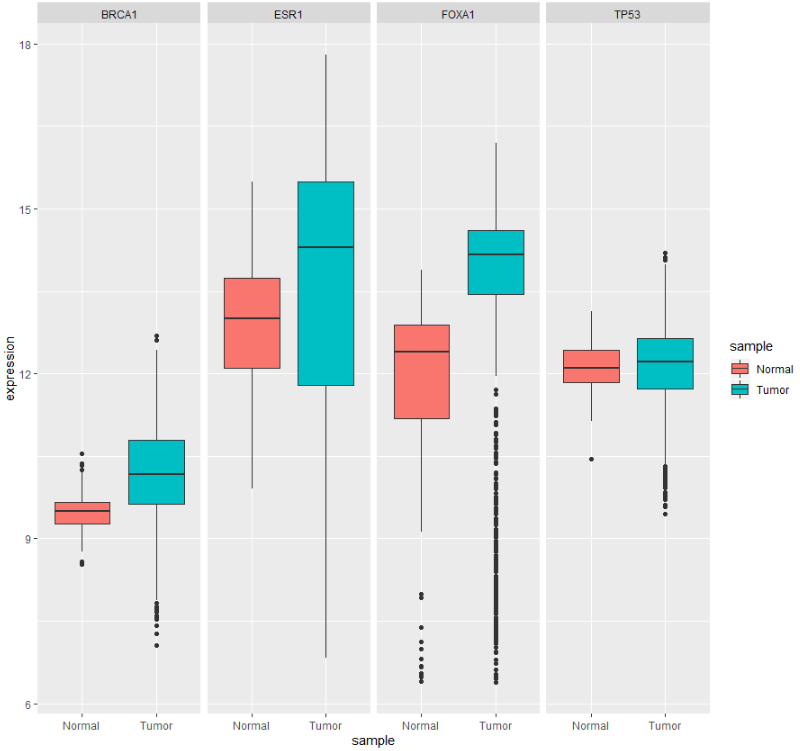pivot_longer的用法就是这么简单，现在我们来探索一下，如何方便地选择多个列。 直接设置cols参数就行，数值和字符串都可以，下面四种方法得到的结果是一样的。

``````data1 <- exprSet %>%
pivot_longer(cols=3:6,
names_to= "gene",
values_to = "expression")

data2 <- exprSet %>%
pivot_longer(cols=-c(1,2),
names_to= "gene",
values_to = "expression")

data3 <- exprSet %>%
pivot_longer(cols=c("BRCA1","TP53","FOXA1","ESR1"),
names_to= "gene",
values_to = "expression")

data4 <- exprSet %>%
pivot_longer(cols=-c("subgroup","sample"),
names_to= "gene",
values_to = "expression")
``````

#### 数据变宽的意义在哪啊？

``````library(tidyr)
data_w <- data1 %>%
pivot_wider( names_from = gene,
values_from = expression)
``````

• values_from，指定那一列数据需要变成更多的列，很明显，现在是expression这一列
• names_from, 指定变成的更多列用什么来指定名称，用gene这一列包含的名称去命名多出来的列 这么一搞，理论上没有问题，但是出现了报错。
``````Warning message:
Values in `expression` are not uniquely identified; output will contain list-cols.
* Use `values_fn = list(expression = list)` to suppress this warning.
* Use `values_fn = list(expression = length)` to identify where the duplicates arise
* Use `values_fn = list(expression = summary_fun)` to summarise duplicates
``````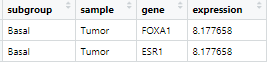pivot_wider不允许这种情况出现，解决方案就是在一开始宽变成的之前增加一列序号作为标识

``````data1 <- exprSet %>%
## 增加一列，序号来表示
mutate(num=seq(1:nrow(.))) %>%
pivot_longer(cols=3:6,
names_to= "gene",
values_to = "expression")
``````

``````data_w <- data1 %>%
pivot_wider( names_from = gene,
values_from = expression)
``````

``````load(file = "BRCAmuts.Rdata")
``````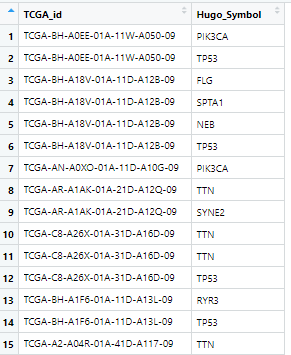group_by和summrise连用后，分组计算就很方便

``````dd <- BRCAmuts %>%
group_by(TCGA_id,Hugo_Symbol)%>%
summarise(num=n())
``````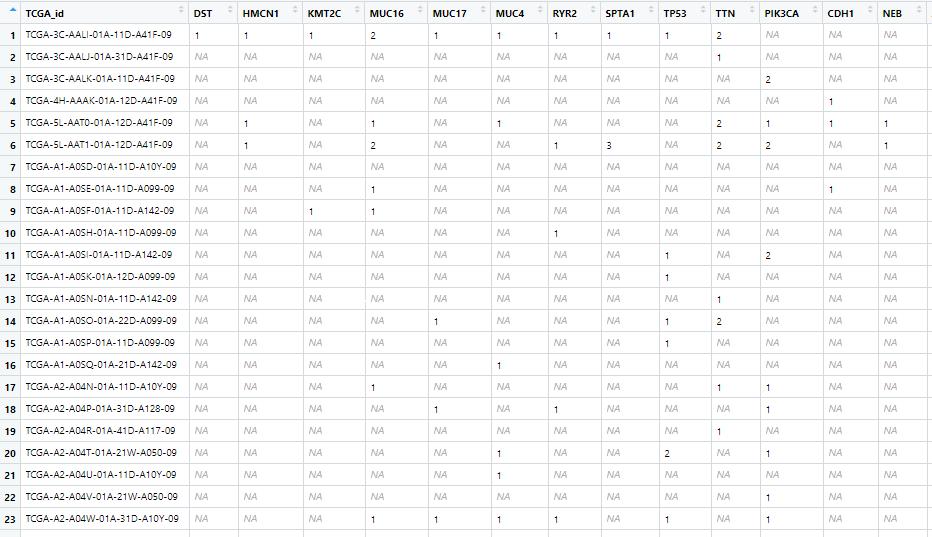然后再见招拆招，看到有很多NA，把他变成0

``````dd1[is.na(dd1)] =0
``````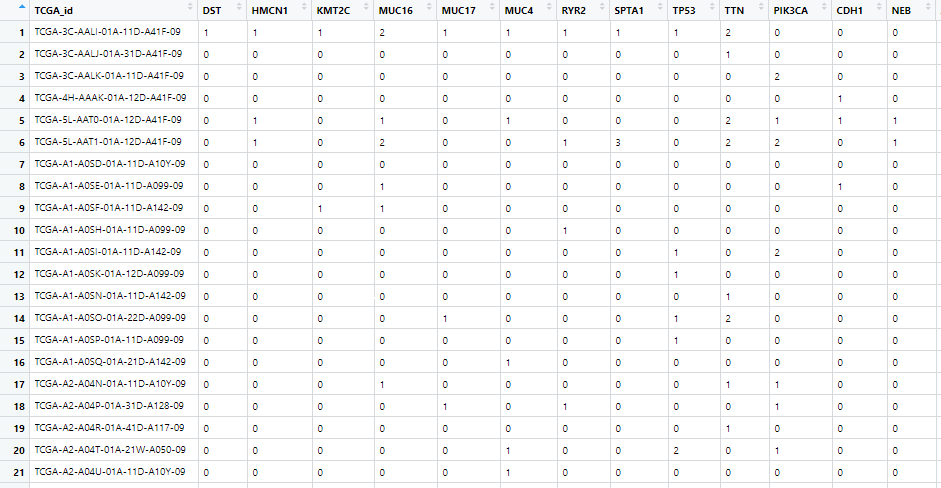当然这个把NA变成0的操作，pivot_wider函数也想到了，可以用values_fill参数来设定，把num这一列最后是NA直接填充为0

``````dd2 <- dd %>%
pivot_wider(names_from =Hugo_Symbol,
values_from = num,
values_fill = list(num = 0)) %>%
ungroup()
``````

``````dd3 <- BRCAmuts %>%
group_by(TCGA_id,Hugo_Symbol)%>%
summarise(num=n()) %>%
pivot_wider(names_from =Hugo_Symbol,
values_from = num,
values_fill = list(num = 0)) %>%
ungroup()
``````

``````dd4 <- reshape2::dcast(BRCAmuts,TCGA_id~Hugo_Symbol)
``````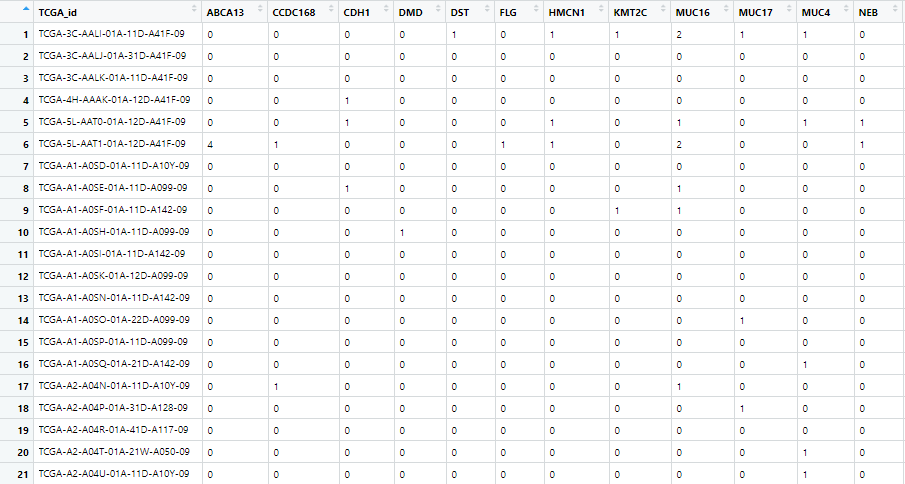]]>